# Sample Size Calculator: Compare Two Proportions – Casagrande, Pike & Smith

Hypothesis:

One sided test: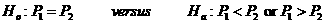Two sided test: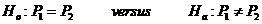Data Input: (Help) (Example)

 Input Results α one sided test two sided test β m P1 P2 N r

Note:

 Variables Descriptions α Significance level 1-β Power of the test P1 Success proportion in arm 1 P2 Success proportion in arm 2 r Ratio of arm 2 to arm 1 m Sample size for arm 1 N Total sample size for arm 1 and 2

Help Aids Top

Application: To calculate the sample sizes needed to detect a difference between two binomial probabilities with specified significance level and power, following hypotheses are usually used:

One sided test:Two sided test:It is shown that over fairly wide ranges of parameter values and ratios of sample sizes, the percentage error which results from using the approximation is no greater than 1%.

Procedure:

1. Enter

a)      value of α, the probability of type I error (choose either one-sided test or two-sided test)

b)      value of β, the probability of type II error, or (1-power) of the test

c)   value of P1, proportion of characteristic present in arm 1

c)      value of P2, proportion of characteristic present in arm 2

d)      value of r, ratio of arm 2 to arm 1

1. Click the button “Calculate” to obtain result sample size for arm 1 m and total sample size N.

Formula:

Define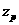be the upper 100(1-p) percentile of the standard normal distribution,

m be the required sample size from the first population,

rm be the required sample size from the second population,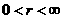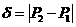,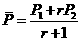and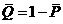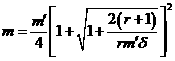(*)

where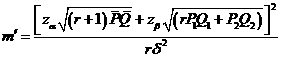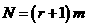Note: (*) is corrected with continuity.

Notations:

α:               The probability of type I error (significance level) is the probability of rejecting the true null hypothesis.

β:               The probability of type II error (1 – power of the test) is the probability of failing to reject the false null hypothesis.

Examples

Example 1:

With significance level α=0.05, equal sample size from two proportions (r=1), the probability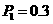and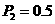are considered sufficiently different to warrant rejecting the hypothesis of no difference. Then the required sample size for two arms to achieve an 80% power (β=0.2) can be determined by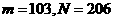.

Reference:

1. Casagrande, Pike and Smith (1978) Biometrics 34: 483-486
2. Fleiss, Tytun and Ury (1980) Biometrics 36: 343-346